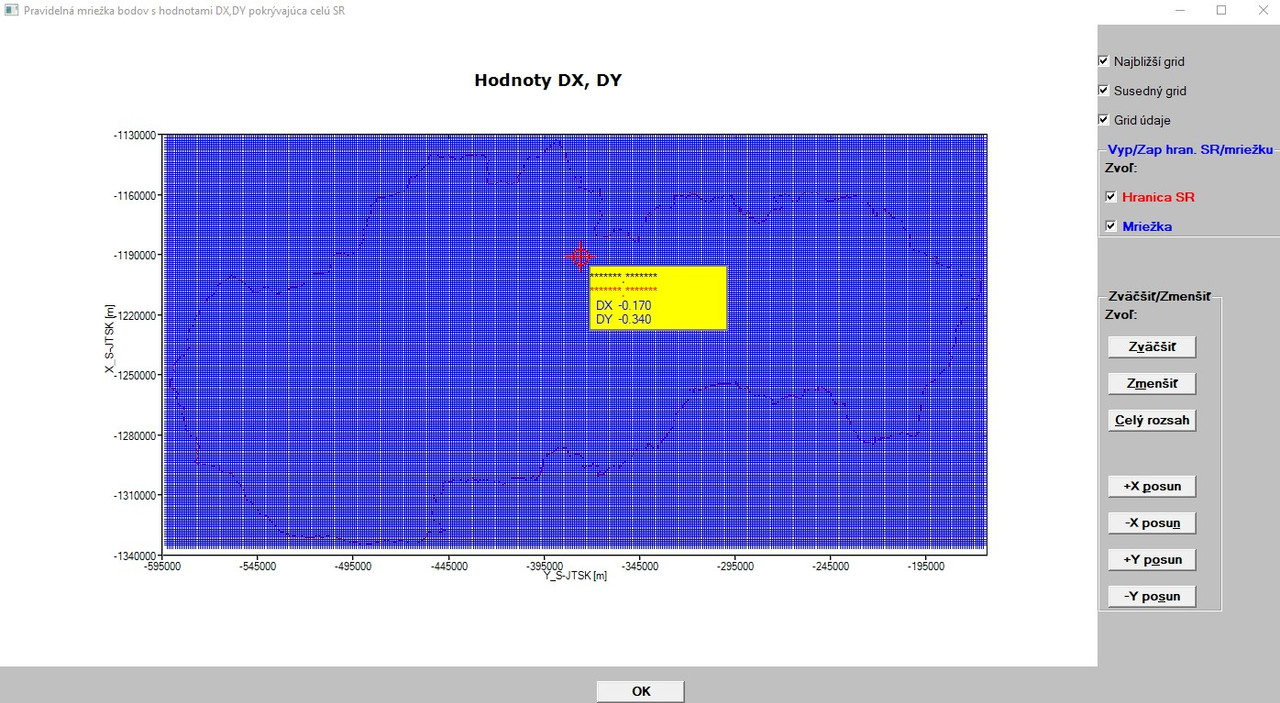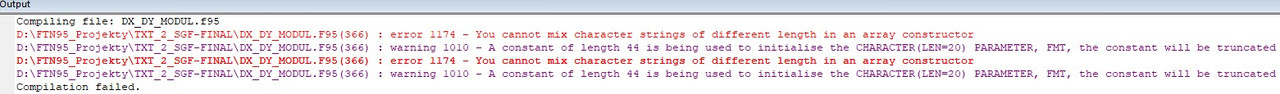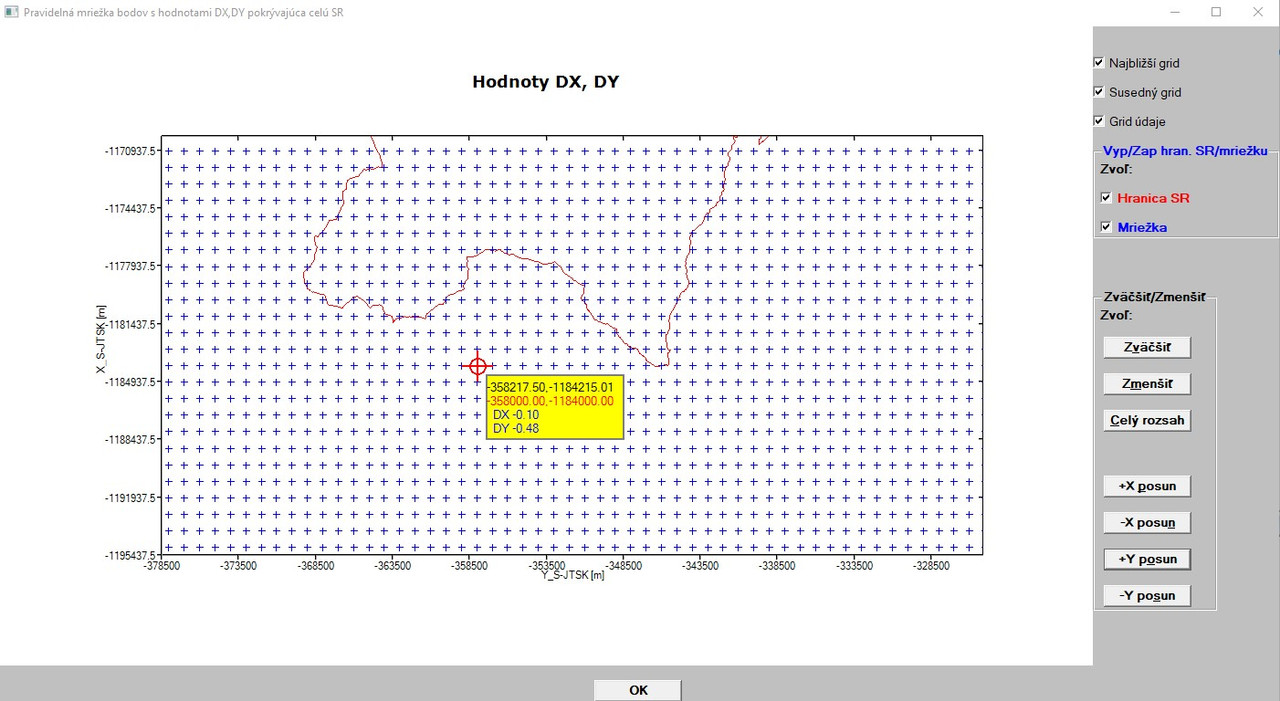%PL - some issues/questions Goto page Previous  1, 2, 3, 4, 5, 6, 7, 8, 9  Next
Author Message
Martin_K

Joined: 09 Apr 2020
Posts: 120Posted: Thu Jun 11, 2020 12:47 pm    Post subject:Ken, just for clarification: I created the binary file containing the binary DX,DY values (and only DX,DY values) separately in a single separate program to have the binary file available for any additional purposes in the future. The binary file was created from the ASCII file containing the X,Y,DX,DY arrays, where only DX,DY values were written to the binary file. So both file have the same number of lines (87312), but binary file contains only DX,DY arrays. So, my graphical program opens a sequential (ASCII) file containing the X,Y, DX, DY arrays to plot them in a graph and upon reading in the whole X,Y arrays from the ASCII file I also open the binary file where I want to read in the corresponding i,j record of DX,DY values determined from the X,Y arrays of the ASCII file to be able to show the X,Y,DX,DY values at the cursor position iwhen moving with the cursor n the graphics.Kenneth_SmithJoined: 18 May 2012
Posts: 378
Location: Hamilton, Lanarkshire, Scotland.Posted: Thu Jun 11, 2020 1:15 pm    Post subject:Example as promised.Kenneth_SmithJoined: 18 May 2012
Posts: 378
Location: Hamilton, Lanarkshire, Scotland.Posted: Thu Jun 11, 2020 1:31 pm    Post subject:Suggest you modify your code to include a write to a direct formatted file immediately after your write to the existing direct unformatted file. You will then be able to check that the data written to the binary file is as expected and looking at the "equivalent" text file. Unformatted direct binary read/write will be faster than formatted, but it's difficult to find the bugs. KenMartin_K

Joined: 09 Apr 2020
Posts: 120Posted: Thu Jun 11, 2020 2:24 pm    Post subject:Thanks Ken! I will review it and try to implement!Kenneth_SmithJoined: 18 May 2012
Posts: 378
Location: Hamilton, Lanarkshire, Scotland.Posted: Thu Jun 11, 2020 2:28 pm    Post subject:OK I see your issue now, and it does not appear to be with the direct file. Minloc should return a value between 1 and 204 for one axis and between 1 and 428 for the other, and there are 204 x 428 = 87,312 records in the binary file. I dont understand how minloc can return a value of 87,312 - unless you have read 87,312 points into the X and Y arrays from your input ascii file? You only need 428 and 204 sets of sequential "axis" coordinates in these arrays. KenMartin_K

Joined: 09 Apr 2020
Posts: 120Posted: Thu Jun 11, 2020 2:59 pm    Post subject:Exactly!

I also expected the value for rows/lines in the interval 1 - 204, since I have 204 different X coordinate values (spacing is 1000m, X goes from North to South, therefore X values are rows/lines, where Xmin = -1335000m and Xmax = - 1132000).

For Y the MINLOC outputs the column value correctly (in the interval between 1 - 428, since I have 428 different Y values, spacing 1000m, Y goes from East to West, therefore Y values are columns, where Ymin = - 592000 and Ymax = -165000).

As I wrote earlier in my today´s post - first I read in the whole array of X,Y values from an ASCII file to be able to plot the X,Y grid (spacing 1000m in each direction) in the graph (either without SK border or with the SK border).

When both X,Y arrays are fully read in, then I use the code:

 Code: riadok_x = minloc(abs(x-xlr),1); stlpec_y = minloc(abs(y-ylr),1)

where X is X array and Y is Y array.

And from that code above I get the variable riadok_x as a value from the interval 1-87312 (incorrect, it should be from the interval 1-204) and the variable stlpec_y from the interval 1-428 (correct)

As I wrote, I created the binary file separately (outside of the graphic program, with another single program, since I do not want to create it (the binary file) each time when I run my graphics program). Binary file contains DX,DY arrays ONLY, it does NOT contain X,Y arrays (I can do it easily, however I thought that both files have the same number of rows/lines (87312), so I could read in the X,Y arrays from the ASCII file and interrogate their I,J position and then look for this I,J position in the binary file containing DX,DY values only.Martin_K

Joined: 09 Apr 2020
Posts: 120Posted: Thu Jun 11, 2020 3:10 pm    Post subject:Supplement information: the 87312 rows in ASCII file look like follows: -1335000 -592000 DX DY -1335000 -591000 DX DY -1335000 -590000 DX DY .... -1335000 -165000 DX DY -1334000 -592000 DX DY -1334000 -591000 DX DY ... -1334000 -165000 DX DY -1333000 -592000 DX DY ... -1333000 -165000 DX DY etc.Kenneth_SmithJoined: 18 May 2012
Posts: 378
Location: Hamilton, Lanarkshire, Scotland.Posted: Thu Jun 11, 2020 4:24 pm    Post subject:So you need to scan your existing input arrays X and Y, removing duplicates and then sorting into ascending order.

Very quick solution:

 Code: program test implicit none integer, parameter :: dp = kind(1.d0) integer, parameter :: n = 20   ! Must be divisble by 4 for this example real(kind=dp) :: x(n), xtmp(n) integer i, k ! Generate some random data with duplicates do i = 1, n, 4   x(i)   =   100.d0*random@() ; x(i+1) =   x(i) ; x(i+2) = - x(i) ; x(i+3) =   100.d0*random@() end do ! print input array with all duplicates print*, 'Input array with duplicates' do i = 1, n   print*, i, x(i) end do call remove_duplicates_and_sort_ascending(x, n, xtmp, k) ! print output array with duplicates removed print* print*, 'Output array with duplicates removed, sorted' print*, 'Number of unique elements = ', k do i = 1, k, 1   print*, i, xtmp(i) end do ! redundant information at end of output array print* print*, 'Redundant information at end of output array' do i = k+1, n, 1   print*, i, xtmp(i) end do end program test subroutine remove_duplicates_and_sort_ascending(array_in, n, array_out, k) implicit none integer, parameter :: dp = kind(1.d0) integer, intent(in)         :: n             ! Number of elements in input array real(kind=dp), intent(in)   :: array_in(1:n) ! Input array real(kind=dp), intent(out)  :: array_out(1:n)! Output array integer, intent(out)        :: k             ! Number of 'usable' elements in output array ! locals real(kind=dp), allocatable :: res(:), d(:) integer,       allocatable :: a(:) integer i     allocate(res(1:n))     res = huge(1.d0)               !!!!! WAS res = 0.d0 ! Remove dulpicates     k = 1     res(1) = array_in(1)     do i=2, n         ! if the number already exist in res check next         if (any( res .eq. array_in(i) )) cycle         ! No match found so add it to the output         k = k + 1         res(k) = array_in(i)     end do !   Copy only unique elements to smaller array d        allocate (d(1:k))     do i = 1, k, 1       d (i) = res(i)     end do !   Create index array a     allocate (a(1:k)) !   Populate array a with pointers to acending locations in d(1:k)        call dsort@(a,d,k)  !!!! DSORT@ sorts the REAL array D by setting pointers from 1 to N in the array A !   Write the first k elements of array_out, containing the unique elements of array_in        array_out = 0.d0        do i = 1, k       array_out(i) = res(a(i))     end do     deallocate(res,d,a) end subroutine  remove_duplicates_and_sort_ascendingKenneth_SmithJoined: 18 May 2012
Posts: 378
Location: Hamilton, Lanarkshire, Scotland.Posted: Thu Jun 11, 2020 10:15 pm    Post subject:You can dispense with the allocatable arrays in the above subroutine using this instead, but unfortunately, it takes longer to run! I was hoping it would be faster.

 Code: subroutine remove_duplicates_and_sort_ascending_2(array_in, n, array_out, k) implicit none integer, parameter :: dp = kind(1.d0) integer, intent(in)         :: n             ! Number of elements in input array real(kind=dp), intent(in)   :: array_in(1:n) ! Input array real(kind=dp), intent(out)  :: array_out(1:n)! Output array integer, intent(out)        :: k             ! Number of 'usable' elements in output array ! locals integer i real(kind=dp) min_val, max_val     array_out = 0.d0     min_val = - huge(1.d0)     max_val = maxval(array_in)     i = 0     do while (min_val .lt. max_val)         i = i+1         min_val = minval(array_in, mask = array_in .gt. min_val)         array_out(i) = min_val     enddo     k = i end subroutine  remove_duplicates_and_sort_ascending_2Martin_K

Joined: 09 Apr 2020
Posts: 120Posted: Fri Jun 12, 2020 5:11 pm    Post subject:Ken,

BIG NEWS! Yesterday, after a football match (we are a group of about 24 recreational football players and we play every Thursday at 18.00 for several years - by the way - yesterday I scored twice and we won 5:4), when we were drinking some bear/wine in a pub I got the following idea how to force my program to do right things when reading in the 87312 line ASCII file with X,Y,DX,DY values and then showing right X,Y,DX,DY values in graphs by means of your LOCATE_I_J_IN_TABLE(I,J,N) function:

 Code: .... .... pocet_roznych_xx = 1        hodnoty_X_Y_DX_DY: do m = 1, pr_subor4               read (8,*) x(m), y(m), dx(m), dy(m)                               if (m.le.428) yy(m) = y(m) ! Limit for 428 different Y values (columns), yy array will be used instead of y array in all subsequent drawing functions                                   if (m.eq.1) then                           ! Limit for 204 different X values (rows/lines), xx array will be used instead of x array in all subsequent drawing functions                   xx(pocet_roznych_xx) = x(m)                                      pocet_roznych_xx = pocet_roznych_xx + 1                                   else                     if (int(x(m)).eq.int(x(m-1))) then                       x(m)=dble(x(m)); x(m-1)=dble(x(m-1))                       cycle                   else                                         xx(pocet_roznych_xx) = x(m)                     pocet_roznych_xx = pocet_roznych_xx + 1                   end if                 end if                             end do hodnoty_X_Y_DX_DY .... ....

The result can be seen here:

[url]And yes, there is still a small error in the display - there are asterisks for X,Y values, which means too small format for the numbers (X,Y) to be dispalyed. But here - although I was looking at your code below 100x - I was unable to get the work done.

 Code: character(len=20), parameter :: fmt(1:2)=(/'(SP,F7.3,1A,F7.3)   ','(SP,2X,A2,1X,F7.3)  '/)

So, first (and sorry for my trivial question in this case, since I was used to use the format statement with a label along with READ/WRITE commands):

I know all other meanings of the code above, but what does it mean 1A?
For example, A2 stands for a character variable with 2 characters, but 1A is what - 1 times a character variable with pre-defined lenght?

Here, in your code above I replaced the F7.3 with F10.2 for Y values (like -496234.22), the second F7.3 I replaced with F11.2 for X values (like -1356852.45) and the third F7.3 with F5.2 for DX/DY values (like -0.45)
as follows:
 Code: character(len=20), parameter :: fmt(1:2)=(/'(SP,F10.2,1A,F11.2)   ','(SP,2X,A2,1X,F5.2)  '/)

but the compiler says:

[url][/url]

Then I tried to adjust the spaces in the FMT to the same length and increased the length of CHARACTER statement - no success.

It is even possible to have different lengths of variables in such way of format defining and if yes how?

So, many thanks Ken for your kind help and inspirations! It helped me fantastically and I am slowly approaching to my final goal, although I still have some new ideas!Kenneth_SmithJoined: 18 May 2012
Posts: 378
Location: Hamilton, Lanarkshire, Scotland.Posted: Fri Jun 12, 2020 7:06 pm    Post subject:Martin,

Good to see you have found a working way around the problem.

 Code: character(len=20), parameter :: fmt(1:2)=(/'(SP,F7.3,1A,F7.3)   ','(SP,2X,A2,1X,F7.3)  '/)

The 1A in fmt(1) above provides the space for the text , in the line below.
 Code: write(output_string(1),fmt(1)) x1r,',',y1r

so you get a comma after the first coordinate.

In this context 1A, A, and A1 all give the same output. For 1A, the one leading 1 means repeat once, and a defines a string of length 1, since there is no number following A.

 Code: character(len=20), parameter :: fmt(1:2)=(/'(SP,F10.2,1A,F11.2)   ','(SP,2X,A2,1X,F5.2)  '/)

Fails for two reasons, firstly the two format descriptors are different lengths, the first is 22 characters long and the second is 20 characters long, so you have to add two blank spaces at the end of the second descriptor. The compiler will still throw an error because the character strings are 22 characters long, but you have defined their length to be 20, so (len=20) must be changed to (len=22).

The code above now becomes:
 Code: character(len=22), parameter :: fmt(1:2)=(/'(SP,F10.2,1A,F11.2)   ','(SP,2X,A2,1X,F5.2)    '/)

It is easier to pick up this type of error when initializing character arrays thus:
 Code: !                                           1234567890123456789012 character(len=22), parameter :: fmt(1:2)=(/'(SP,F10.2,1A,F11.2)   ', &                                            '(SP,2X,A2,1X,F5.2)    '/)

KenMartin_K

Joined: 09 Apr 2020
Posts: 120Posted: Sat Jun 13, 2020 12:09 am    Post subject:Ken - many thanks! Now it works as expected! [url] https://postimg.cc/Yh3BtW9m][/url] Later - more! Do you have some info, when the problem with SYMBOL=12 and SYMBOL=13 will be fixed? MartinJohn-SilverJoined: 30 Jul 2013
Posts: 1451
Location: Aerospace ValleyPosted: Sat Jun 13, 2020 11:19 am    Post subject:Paul, at the same time as yougive feedback to Martin about the symbols, could you reply please to my comment on 7th June (see p.6 of thread) where I asked about the proximity of the axes labels to the tick labels. Thanks. Martin ... do you us default 'margins' in your plot above or have you specified a particular size ? I ask because although the '% white space' of your plot is not enormous, it could imo be optimised further to give the best possible data visualisation. I's one of the key 'recommendation in publictions concerning data presentation and visibility._________________''Computers (HAL and MARVIN excepted) are incredibly rigid. They question nothing. Especially input data.Human beings are incredibly trusting of computers and don't check input data. Together cocking up even the simplest calculation ..."Martin_K

Joined: 09 Apr 2020
Posts: 120Posted: Sat Jun 13, 2020 12:31 pm    Post subject:John,

here is a part of the code I use for plotting:

 Code: ... CALL winop@('%pl[native]') CALL winop@('%pl[scale=linear]') CALL winop@('%pl[fixed_aspect]') CALL winop@('%pl[x_sigfigs=7]') CALL winop@('%pl[y_sigfigs=7]') CALL winop@('%pl[link=lines,symbol=0]') CALL winop@('%pl[link=none,symbol=12]') CALL winop@('%pl[title="Hodnoty DX, DY [m]"]') CALL winop@('%pl[x_axis="Y_S-JTSK [m]"]') CALL winop@('%pl[y_axis="X_S-JTSK [m]"]') CALL winop@('%pl[x_array]') CALL winop@('%pl[independent]') CALL winop@('%pl[colour=red,colour=blue,n_graphs=2,frame,etched]') iw=winio@('%`^pl[full_mouse_input]&',gw,gh,npoints,y_hr,x_hr,y,x,handle_pl,pl_cb) ....

I would also like to have more control over axes captions (in my case Y_S-JTSK [m] for X axis and X_S-JTSK [m] for Y axis, since they practically touch the numbers above them, what is not well arranged. I would like to put them more to the bottom and more to the left (to have visible spacing between the captions and numbers) and put both in BOLD font (and maybe also in another colour than black), but I found nothing in the help how to do it.

Ken,

still once more back to your explanation with FMT issue. As you advised, I changed your code as follows (I also changed A2 to A3 in FMT(2)
to have the text DX= and DY= in the box rectangle in the graphics):

 Code: character(len=22), parameter :: fmt(1:2)=(/'(SP,F10.2,1A,F11.2)   ','(SP,2X,A3,1X,F5.2)    '/)

While I understand that FMT(1) is 22 characters long (SP character (plus sign)+10+11=22), I see the FMT(2) as 9 characters long (SP+2x+1x+5=9). When I add to them the 4 trailing blank spaces I get 13.

How can I get the 22 character length for FMT(2)?

MartinKenneth_SmithJoined: 18 May 2012
Posts: 378
Location: Hamilton, Lanarkshire, Scotland.Posted: Sat Jun 13, 2020 1:57 pm    Post subject:This string is 22 characters long:

 Code: '(SP,F10.2,1A,F11.2)   '

and this string is also 22 characters long:

 Code: '(SP,2X,A3,1X,F5.2)    '

This is totally independent of the actual number of characters produced by the read or write statements that refer to these strings as format codes.

You can now reduce len to 19.

 Code: character(len=19), parameter :: fmt(1:2)=(/'(SP,F10.2,1A,F11.2)',&                                            '(SP,2X,A3,1X,F5.2) '/)

KenDisplay posts from previous: All Posts1 Day7 Days2 Weeks1 Month3 Months6 Months1 Year Oldest FirstNewest First
 All times are GMT + 1 HourGoto page Previous  1, 2, 3, 4, 5, 6, 7, 8, 9  Next Page 7 of 9